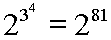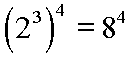# Expression Evaluation, Parentheses

Basically, parentheses are used in expressions to change the natural order of precedence among the operators. For example, consider this expression:

3 + 4 * 5

Since multiplication has precedence over addition, we know that this expression will be evaluated as follows: Four times five is twenty; twenty plus three is twenty-three. Or, in notation:

3 + 4 * 5

3 + 20

23

But what if you want to add the three and the four first, and then multiply by five? How do you then set up the notation? You use parentheses, like this:

(3 + 4) * 5

One always evaluates the expression inside of parentheses first. So, for the above situation three is added to four to get seven, and then seven is multiplied with five to get thirty-five. Again, in notation:

(3 + 4) * 5

7 * 5

35

The expression inside of the parentheses may have its own precedence for operations. For example:

(2 + 4 * 6) * 3

Would work this way: Inside the parentheses multiplication has precedence over addition, so four would be multiplied by six to get twenty-four, and then two would be added to twenty-four to get twenty-six. That is the value inside of the parentheses. This value, twenty-six would then be multiplied by three to finally get a value of seventy-eight. In notation:

(2 + 4 * 6) * 3

(2 + 24) * 3

26 * 3

78

Notice, regarding an example which we have seen before:

-42 = -16

That is, the negative of the second power of four is negative sixteen. But by using parentheses we can state:

(-4)2 = 16

That is, the second power of negative four is positive sixteen, or negative four raised to the second power is positive sixteen.

Here is another example from before:Notice how parentheses changes things; here, it is the third power of two which receives its value first, making a value of eight, and then this value is raised to the fourth power:As we saw before, 281 = 2,417,851,639,229,258,349,412,352. But 84 = 4,096. Certainly changing the natural order of the operators with parentheses changes quite a bit here.

Sometimes parentheses have a use that does not look much like they are being used to change the order of operator precedence. They can be used to group terms even if this grouping does not change the order of evaluation. For example, the parentheses are not really needed here, since multiplication automatically has precedence over addition:

4 + (5 * 3)

However, they do no harm, and, perhaps, the author wants to show some special feature in a problem where the five times the three forms a unit of thought currently under discussion, so, it is emphasized this way.

Where are you?

Here: Parentheses

Custom Search